Next: Budan-Fourier method Up: Descartes Lemma Previous: Mathematical background   Contents

Implementation

The syntax of the procedure is:

INT Descartes_Lemma_Interval(int Degree,VECTOR &Coeff)
with:
• Degree: degree of the polynomial
• Coeff: the Degree+1 coefficients of the polynomial in increasing degree
This procedure returns the number of positive real roots up to an even number. If the procedure returns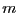the number of positive roots is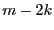with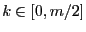.

There is an implementation of this method for interval polynomial. Here it is necessary to introduce an additional parameter to indicate the confidence we have in the result. The procedure is implemented as:

INT Descartes_Lemma_Interval(int Degree,INTERVAL_VECTOR &Coeff,int *Confidence);
Confidence is a quality index:
• 1: the result is exact, for all polynomials in the set the number of positive real roots is•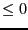: the number of positive real roots for all the polynomials in the set is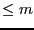Similar algorithms for negative roots are available:

INT Descartes_Lemma_Negative_Interval(int Degree,VECTOR &Coeff)
INT Descartes_Lemma_Negative_Interval(int Degree,INTERVAL_VECTOR &Coeff,int *Confidence)

Jean-Pierre Merlet 2012-12-20